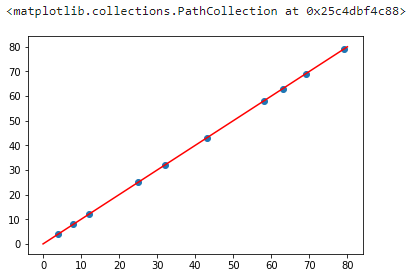# 机器学习|多项式回归算法详解 (Python 语言描述)

## 多项式回归介绍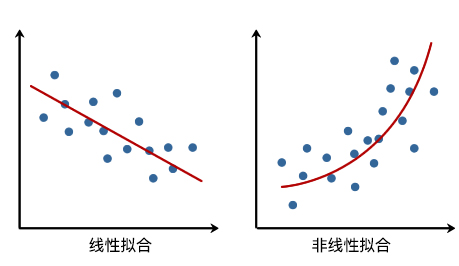## 多项式回归基础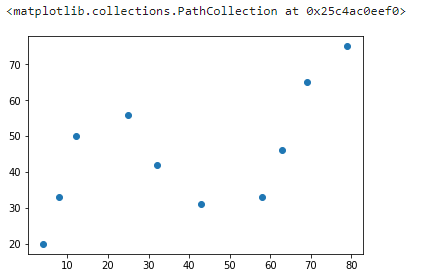### 实现 2 次多项式拟合

$$y(x, w) = w_0 + w_1x + w_2x^2 +…+w_mx^m = \sum\limits_{j=0}^{m}w_jx^j \tag{1}$$

$$y(x, w) = w_0 + w_1x + w_2x^2= \sum\limits_{j=0}^{2}w_jx^j \tag{2}$$

$$y(x) = 37 - 0.26x + 0.0080x^2 \tag{3}$$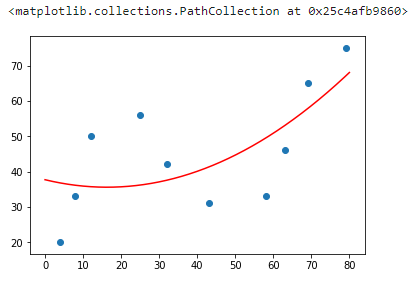### 实现 N 次多项式拟合

$$y(x) = 0.0080x^2 - 0.26x + 37\tag{4}$$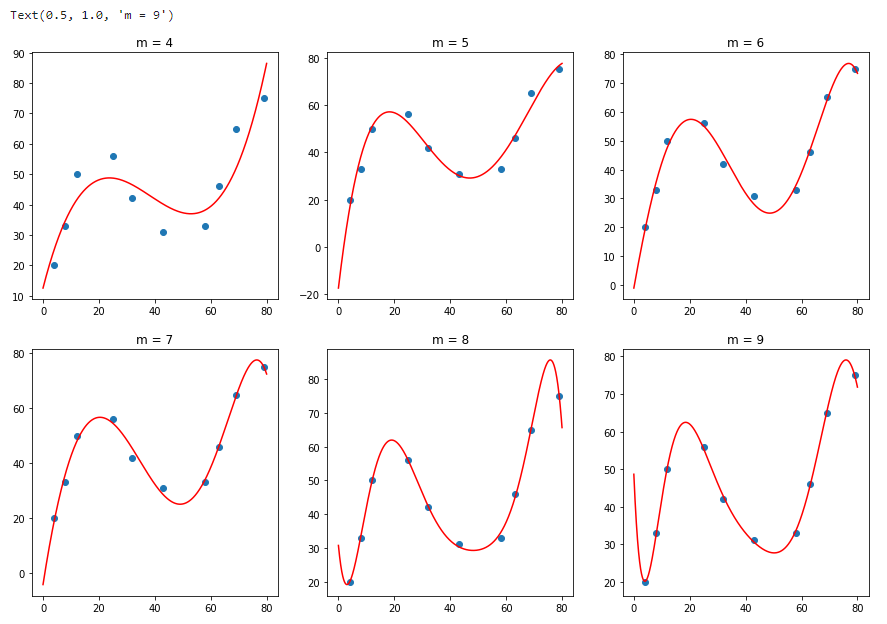### 使用 scikit-learn 进行多项式拟合$$\mathbf{X} = \left [ X_{1}, X_{2}, X_{1}^2, X_{1}X_{2}, X_{2}^2 \right ] \tag{5c}$$

• degree: 多项式次数，默认为 2 次多项式
• interaction_only: 默认为 False，如果为 True 则产生相互影响的特征集。
• include_bias: 默认为 True，包含多项式中的截距项。

• fit_intercept: 默认为 True，计算截距项。
• normalize: 默认为 False，不针对数据进行标准化处理。
• copy_X: 默认为 True，即使用数据的副本进行操作，防止影响原数据。
• n_jobs: 计算时的作业数量。默认为 1，若为 -1 则使用全部 CPU 参与运算。